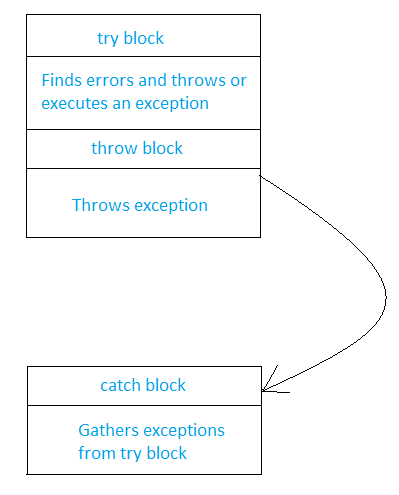# C++ Program to Handle the Unchecked Exceptions

Exceptions are run-time errors or abnormal conditions that a program may encounter during execution.

Examples:

• Division by zero
• Running out of memory
• Running out of disk space

Types of Exceptions:

• Synchronous Exceptions: The exceptions which occur during the program execution due to some fault in the input data. For example, Errors such as overflow, and division by zero.
• Asynchronous Exceptions: The exceptions caused by events or faults unrelated (extended) to the program and beyond the control of the program. For example Keyboard failures, and hardware disk failures.

The exception handling in  C++ is designed to handle only synchronous exceptions in a program. The goal of exception handling is to create a routine that checks and sends an exceptional condition in order to execute suitable code. The procedure needs to carry out the following responsibilities:

• Detect the problem(Hit the exception)
• Tells about error detection(throw the exception)
• Receive error information(Catch the exception)
• Take corrective action(Handle the exception)

The keywords try, throw, and catch. The keyword try is used to preface a block of code which may result in exceptions.

Syntax of try statement:

```try{
statement1;
statement2;
}```

When an exception is found, it is thrown using a throw statement in the try block.

Syntax of the throw statement

```throw(excep);
throw excep;
throw;// re-throwing of an exception```

A catch block is defined by the keyword ‘catch‘ the exception and handles it accordingly. The catch block that catches an exception must immediately follow the try block of the exception.

Syntax of catch statement:

```try{
statement1;
statement2;
}
catch (argument)
{
statement3;// action to be taken
}```

When an exception is found, the execution of the catch block starts. The catch statement may or may not contains an argument of exception type, it is optional. When an argument is declared in the catch, the argument can be used in the catch block. After the execution of the catch block, the lines inside the blocks are executed. In case no exception is found, the catch block is ignored and if a mismatch is found, the program is finished.## C++

 `// C++ program to illustrate  ` `// division by zero exception ` `#include ` `using` `namespace` `std; ` ` `  `int` `main() ` `{ ` ` `  `    ``int` `Gfg1 = 9, Gfg2 = 0; ` ` `  `    ``// try block starts here ` `    ``try` `{ ` `        ``if` `(Gfg2 != 0) ` `            ``cout << Gfg1 / Gfg2; ` `        ``else` `            ``throw` `Gfg2; ``// throwing if Gfg2 is zero ` `    ``} ` `    ``// catch block starts here ` `    ``catch` `(``int` `i) { ` `       `  `        ``/// if b is zero means division is done 0 hence ` `        ``// thrown from try block and catch ` `        ``cout << ``"Division by zero: "` `<< i << endl; ` `    ``} ` ` `  `    ``return` `0; ` `}`

Output:

`Division by zero: 0`

## C++

 `// C++ program to demonstrate  ` `// array index out of bounds ` `// exception ` `#include ` `using` `namespace` `std; ` ` `  `int` `main() ` `{ ` `    ``// initialize an array of  ` `    ``// size 5 with numbers from 1 to ` `    ``// 5 ` `    ``int` `a = { 1, 2, 3, 4, 5 }, i; ` `   `  `    ``// try block starts here ` `    ``try` `{ ` `        ``i = 0; ``// initialize i with 0 ` `               ``// loop for printing value of array till its ` `               ``// size 5 ` `        ``while` `(1) { ` `            ``if` `(i != 5) { ` `                ``cout << a[i] << endl; ` `                ``i++; ` `            ``} ` `            ``// if i go beyond 5 then throw exception ` `            ``else` `                ``throw` `i; ` `        ``} ` `    ``} ` `    ``// catch the exception ` `    ``catch` `(``int` `i) { ` `        ``cout << ``"Array Index out of Bounds Exception: "` `<< i ` `             ``<< endl; ` `    ``} ` `    ``return` `0; ` `}`

Output:

```1
2
3
4
5
Array Index out of Bounds Exception: 5```

## C++

 `// C++ program to throw multiple ` `// exceptions and define ` `// multiple catch statement ` `#include ` `using` `namespace` `std; ` ` `  `// function to check if number is ` `// positive, negative or zero ` `void` `num(``int` `k) ` `{ ` `    ``try` `{ ` `        ``if` `(k == 0) ` `            ``throw` `k; ``// throwing int value ` `       `  `        ``else` `if` `(k > 0) ` `            ``throw` `'P'``; ``// throwing char value ` `       `  `        ``else` `if` `(k < 0) ` `            ``throw` `1.0; ``// throwing double value ` `       `  `        ``cout << ``"*** end of try block ***\n"``; ` `    ``} ` `    ``// catching char value ` `    ``catch` `(``char` `g) { ` `        ``cout << ``"Caught a positive value \n"``; ` `    ``} ` `    ``// catching integer value ` `    ``catch` `(``int` `j) { ` `        ``cout << ``"caught an null value \n"``; ` `    ``} ` `    ``// catching double value ` `    ``catch` `(``double` `f) { ` `        ``cout << ``"Caught a Negative value \n"``; ` `    ``} ` ` `  `    ``cout << ``"*** end of try catch ***\n \n"``; ` `} ` `int` `main() ` `{ ` `    ``cout << ``"Demo of Multiple catches"` `<< endl; ` `    ``num(0); ``// function call for zero ` `    ``num(5); ``// function call for positive ` `    ``num(-1); ``// function call for negative ` `    ``return` `0; ` `}`

Output:

```Demo of Multiple catches
caught an null value
*** end of try catch ***

Caught a positive value
*** end of try catch ***

Caught a Negative value
*** end of try catch ***```

Whether you're preparing for your first job interview or aiming to upskill in this ever-evolving tech landscape, GeeksforGeeks Courses are your key to success. We provide top-quality content at affordable prices, all geared towards accelerating your growth in a time-bound manner. Join the millions we've already empowered, and we're here to do the same for you. Don't miss out - check it out now!

Previous
Next